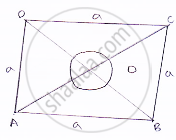# A Rhombus Sheet, Whose Perimeter is 32 M and Whose One Diagonal is 10 M Long, is Painted on Both Sides at the Rate of Rs 5 per M2. Find the Cost of Painting. - Mathematics

A rhombus sheet, whose perimeter is 32 m and whose one diagonal is 10 m long, is painted on both sides at the rate of Rs 5 per m2. Find the cost of painting.

#### Solution

Given that,
Perimeter of a rhombus = 32 m
We know that,
Perimeter of rhombus = 4 × side
⇒ 49 = 32m
⇒ a = 8 m
Let AC = 10 = OA = 1/2𝐴𝐶
= 1/2×10
= 5𝑚By using Pythagoras theorem:

∴OB^2=AB^2-OA^2

⇒OB=sqrt(AB^2-OA^2)

⇒OB=sqrt(8^2-5^2)

⇒OB=sqrt(64-25)

⇒OB=sqrt(39m)

Now, BD = 2OB = 2√39𝑚

∴ Area of sheet = 1/2×𝐵𝐷×𝐴𝐶=1/2×2sqrt(39)xx10sqrt(39)m^2

∴ Cost of printing on both sides at the rate of Rs 5 per m^2 = Rs 2 ×10sqrt(39)×5
= Rs. 625.00

Concept: Area of a Triangle by Heron's Formula
Is there an error in this question or solution?

#### APPEARS IN

RD Sharma Mathematics for Class 9
Chapter 17 Heron’s Formula
Exercise 17.2 | Q 6 | Page 19

Share